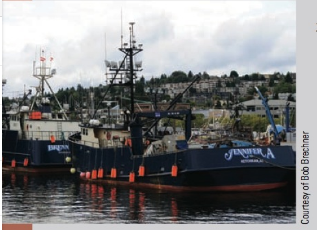Chapter 1, Problem 27AT### Contemporary Mathematics for Busin...

8th Edition
Robert Brechner + 1 other
ISBN: 9781305585447

#### Solutions

Chapter
Section### Contemporary Mathematics for Busin...

8th Edition
Robert Brechner + 1 other
ISBN: 9781305585447
Textbook Problem

# Last week the More Joy, a commercial fishing boat in Alaska, brought in 360 pounds of salmon, 225 pounds of halibut, and 570 pounds of cod. At the dock, the catch was sold to Pacific Seafood Wholesalers. The salmon brought $3 per pound; the halibut,$4 per pound; and the cod, $5 per pound. If fuel and crew expenses amounted to$1,644, how much profit did Captain Bob make on this trip?Alaskan Fishing boats According to the Alaska Department of Fish & Game, Alaska supports one of the most productive commercial fishing economies in the world, with more than 9,600 licensed vessels as well as 20,500 licensed crewmembers.Alaskan fishermen typically receive well over $1 billion for their catch, while the value of Alaskan seafood sold at first wholesale easily tops$2 billion per year.

To determine

To calculate: How much profit did captain make on the trip in which he brought in 360 pounds of salmon, 225 pounds of halibut and 570 pound of cod. And at the dock sold the salmon at $3 per pound, the halibut at$4 per pound and the cod at $5 per pound whereas apart from this the fuel and crew expenses are amounted to$1,644.

Explanation

Given Information:

The captain brought in 360 pounds of salmon, 225 pounds of halibut and 570 pound of cod.

The captain sold the salmon at $3 per pound, the halibut at$4 per pound and the cod at $5 per pound. The fuel and crew expenses are amounted to$1,644.

Formula used:

Steps for multiplying whole numbers.

Step 1: Write down the factors such that the place values line up in columns.

Step 2: Multiply every digit of the multiplier with the multiplicand, starting with the units. Each multiplication gives a partial product whose unit digit appears under the same column that is the corresponding digit of the multiplier.

Step 3: Now in each column, add the digits of the partial products, starting with the unit’s column on the right.

Step 1: Line up the place values by writing the whole numbers in column- units, tens, hundreds, thousands, and so on.

Step 2: Starting from the right with the units column, add the digits in each column.

Step 3: Write the units digit and carry the tens digit to the top of the next column to the left, when the total in column is greater than 9.

Steps for subtracting whole number,

Step 1: Line up the place values by writing the whole numbers in column- units, tens, hundreds, thousands, and so on.

Step 2: Starting from the right with the units column, subtract the digits in each column.

Step 3: A digit must be borrowed from the column to the left of the working column, if the column cannot be subtracted.

Calculation:

Consider the provided data,

The captain brought in 360 pounds of salmon and the captain sold the salmon at $3 per pound. Therefore, the profit through salmon will be, pounds of fish×price per pound So, the expression becomes, 360× 3 ¯ Consider the provided expression, 360× 3 ¯ Multiply the multiplier ‘3’ with the multiplicand: 360× 3 1080 ¯ Therefore, the profit through salmon is$1080.

Now consider the following data,

The captain brought 225 pounds of halibut and the captain sold the halibut at $4 per pound Therefore, the profit through halibut will be, pounds of fish×price per pound So the expression becomes, 225× 4 ¯ Consider the provided expression, 225× 4 ¯ Multiply the multiplier ‘4’ with the multiplicand. 225× 4 900 ¯ Therefore, the profit through halibut is$900.

Now consider the data,

The captain brought in 570 pound of cod and the captain sold the cod at $5 per pound. Therefore, the profit through cod will be, pounds of fish×price per pound So, the expression becomes, 570× 5 ¯ Consider the provided expression, 570× 5 ¯ Multiply the multiplier ‘5’ with the multiplicand: 570× 5 2850 ¯ Therefore, the profit through cod is$2,850.

Now, the total amount made by the captain through the fish will be,

total price of salmon+total price of halibut+total price of cod

So, the expression becomes,

2850      1080+     900               ¯

Consider the operation, and line up the place values:

2850      1080+     900               ¯

Now, add the digits in each column starting from the right with the unit’s column

### Still sussing out bartleby?

Check out a sample textbook solution.

See a sample solution

#### The Solution to Your Study Problems

Bartleby provides explanations to thousands of textbook problems written by our experts, many with advanced degrees!

Get Started

#### Find more solutions based on key concepts